International
Tables for
Crystallography
Volume B
Reciprocal space
Edited by U. Shmueli

International Tables for Crystallography (2006). Vol. B. ch. 1.2, p. 11   | 1 | 2 |

## Section 1.2.5.1. Nuclear scattering

P. Coppensa*

aDepartment of Chemistry, Natural Sciences & Mathematics Complex, State University of New York at Buffalo, Buffalo, New York 14260-3000, USA
Correspondence e-mail: coppens@acsu.buffalo.edu

#### 1.2.5.1. Nuclear scattering

| top | pdf |

The scattering of neutrons by atomic nuclei is described by the atomic scattering length b, related to the total cross section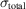by the expression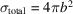. At present, there is no theory of nuclear forces which allows calculation of the scattering length, so that experimental values are to be used. Two types of nuclei can be distinguished (Squires, 1978). In the first type, the scattering is a resonance phenomenon and is associated with the formation of a compound nucleus (consisting of the original nucleus plus a neutron) with an energy close to that of an excited state. In the second type, the compound nucleus is not near an excited state and the scattering length is essentially real and independent of the energy of the incoming neutron. In either case, b is independent of the Bragg angle θ, unlike the X-ray form factor, since the nuclear dimensions are very small relative to the wavelength of thermal neutrons.

The scattering length is not the same for different isotopes of an element. A random distribution of isotopes over the sites occupied by that element leads to an incoherent contribution, such that effectively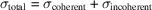. Similarly for nuclei with non-zero spin, a spin incoherent scattering occurs as the spin states are, in general, randomly distributed over the sites of the nuclei.

For free or loosely bound nuclei, the scattering length is modified by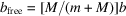, where M is the mass of the nucleus and m is the mass of the neutron. This effect is of consequence only for the lightest elements. It can, in particular, be of significance for hydrogen atoms. With this in mind, the structure-factor expression for elastic scattering can be written as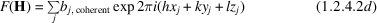by analogy to (1.2.4.2b).

### ReferencesSquires, G. L. (1978). Introduction to the theory of thermal neutron scattering. Cambridge University Press.Google Scholar